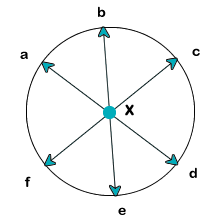Home > Pre-Algebra > Area > How to Find the Area of a Circle

## How to Find the Area of a Circle

#### Introduction

A circle is a geometric figure which is the set of all points in a plane that are equidistant from a given point called its center.The distance between any point of the circle and the center is called the radius. The distance from one side of the circle to another that passes through the center is called the diameter. The diameter is always twice as long as the radius.

#### Terms

Area - A quantity that expresses the extent of a two-dimensional shape.
Chord - A line segment whose endpoints lie on the circle.
Circle - A geometric figure which is the set of all points in a plane that are equidistant from a given point called its center.
Circumference - The distance around the circle.
Diameter - A chord that passes through the center of the circle. Equal to twice the radius.
Pi - A constant that describes the ratio between the circumference and diameter of a circle. It is equal to 3.14159..., but typically rounded to 3.14 for calculation.
Radius - The distance between of a point that lie on the circle from the center. Equal to half the diameter.

## Lesson

Area of a Circle Formula:

$ext{Area} = pi * r^{2}$

If the radius is given as 4, we can substitute it into the equation above like this:

$ext{Radius} = 4$

$ext{Area} = pi * (4)^{2}$

$ext{Area} = pi * 16$

$ext{Area} = 3.14 * 16 = 50.24$

If we are given a diameter of 10 instead of the radius, we need to calculate the radius before we can use the formula. We know that the radius is always half the diameter ($ext{Radius} = ext{Diameter} div 2$), so we can plug in 10 as the diameter and calculate the radius:

$ext{Radius} = dfrac{10}{2}$

$ext{Radius} = 5$

Then we are able to use the formula above:

$ext{Radius} = 5$

$ext{Area} = pi * (5)^{2}$

$ext{Area} = pi * 25$

$ext{Area} = 3.14 * 25 = 78.5$

## Examples

#### Area of a Circle Given Diameter (Whole Numbers)

$\text{Radius} = \dfrac{\text{Diameter}}{\text{2}}$
$\text{Radius} = \dfrac{20}{2}$
$\text{Radius} = 10$
$\text{Area} = \pi * \text{(Radius)}^{2}$
$\text{Area} = 3.14 * 100$
$\text{Area} = 314$

#### Area of a Circle Given Radius (Whole Numbers)

$\text{Area} = \pi * \text{(Radius)}^{2}$
$\text{Area} = 3.14 * 64$
$\text{Area} = 200.96$

#### Area of a Circle Given Diameter (Decimals)

$\text{Radius} = \dfrac{\text{Diameter}}{\text{2}}$
$\text{Radius} = \dfrac{15.64}{2}$
$\text{Radius} = 7.82$
$\text{Area} = \pi * \text{(Radius)}^{2}$
$\text{Area} = 3.14 * 61.1524$
$\text{Area} = 192.02$

#### Area of a Circle Given Radius (Decimals)

$\text{Area} = \pi * \text{(Radius)}^{2}$
$\text{Area} = 3.14 * 56.5504$
$\text{Area} = 177.57$